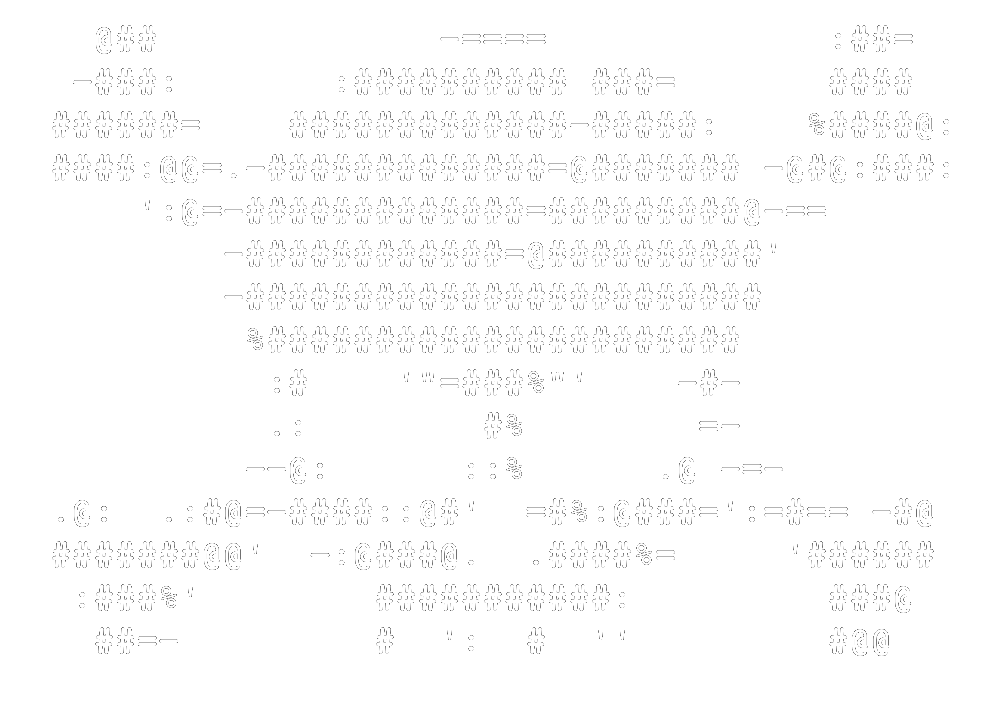``` HyperSec.xyz Cryptography: Vigenere Hashes: Identifier Hash MD5 Hash SHA1 Hash SHA256 Hash SHA512 Password attacks: Wordlists OSINT: Email search Network: IP geolocator Extras: Unix tips PAX Dicts Main site var MD5 = function(d){var r = M(V(Y(X(d),8*d.length)));return r.toLowerCase()};function M(d){for(var _,m="0123456789ABCDEF",f="",r=0;r<d.length;r++)_=d.charCodeAt(r),f+=m.charAt(_>>>4&15)+m.charAt(15&_);return f}function X(d){for(var _=Array(d.length>>2),m=0;m<_.length;m++)_[m]=0;for(m=0;m<8*d.length;m+=8)_[m>>5]|=(255&d.charCodeAt(m/8))<<m%32;return _}function V(d){for(var _="",m=0;m<32*d.length;m+=8)_+=String.fromCharCode(d[m>>5]>>>m%32&255);return _}function Y(d,_){d[_>>5]|=128<<_%32,d[14+(_+64>>>9<<4)]=_;for(var m=1732584193,f=-271733879,r=-1732584194,i=271733878,n=0;n<d.length;n+=16){var h=m,t=f,g=r,e=i;f=md5_ii(f=md5_ii(f=md5_ii(f=md5_ii(f=md5_hh(f=md5_hh(f=md5_hh(f=md5_hh(f=md5_gg(f=md5_gg(f=md5_gg(f=md5_gg(f=md5_ff(f=md5_ff(f=md5_ff(f=md5_ff(f,r=md5_ff(r,i=md5_ff(i,m=md5_ff(m,f,r,i,d[n+0],7,-680876936),f,r,d[n+1],12,-389564586),m,f,d[n+2],17,606105819),i,m,d[n+3],22,-1044525330),r=md5_ff(r,i=md5_ff(i,m=md5_ff(m,f,r,i,d[n+4],7,-176418897),f,r,d[n+5],12,1200080426),m,f,d[n+6],17,-1473231341),i,m,d[n+7],22,-45705983),r=md5_ff(r,i=md5_ff(i,m=md5_ff(m,f,r,i,d[n+8],7,1770035416),f,r,d[n+9],12,-1958414417),m,f,d[n+10],17,-42063),i,m,d[n+11],22,-1990404162),r=md5_ff(r,i=md5_ff(i,m=md5_ff(m,f,r,i,d[n+12],7,1804603682),f,r,d[n+13],12,-40341101),m,f,d[n+14],17,-1502002290),i,m,d[n+15],22,1236535329),r=md5_gg(r,i=md5_gg(i,m=md5_gg(m,f,r,i,d[n+1],5,-165796510),f,r,d[n+6],9,-1069501632),m,f,d[n+11],14,643717713),i,m,d[n+0],20,-373897302),r=md5_gg(r,i=md5_gg(i,m=md5_gg(m,f,r,i,d[n+5],5,-701558691),f,r,d[n+10],9,38016083),m,f,d[n+15],14,-660478335),i,m,d[n+4],20,-405537848),r=md5_gg(r,i=md5_gg(i,m=md5_gg(m,f,r,i,d[n+9],5,568446438),f,r,d[n+14],9,-1019803690),m,f,d[n+3],14,-187363961),i,m,d[n+8],20,1163531501),r=md5_gg(r,i=md5_gg(i,m=md5_gg(m,f,r,i,d[n+13],5,-1444681467),f,r,d[n+2],9,-51403784),m,f,d[n+7],14,1735328473),i,m,d[n+12],20,-1926607734),r=md5_hh(r,i=md5_hh(i,m=md5_hh(m,f,r,i,d[n+5],4,-378558),f,r,d[n+8],11,-2022574463),m,f,d[n+11],16,1839030562),i,m,d[n+14],23,-35309556),r=md5_hh(r,i=md5_hh(i,m=md5_hh(m,f,r,i,d[n+1],4,-1530992060),f,r,d[n+4],11,1272893353),m,f,d[n+7],16,-155497632),i,m,d[n+10],23,-1094730640),r=md5_hh(r,i=md5_hh(i,m=md5_hh(m,f,r,i,d[n+13],4,681279174),f,r,d[n+0],11,-358537222),m,f,d[n+3],16,-722521979),i,m,d[n+6],23,76029189),r=md5_hh(r,i=md5_hh(i,m=md5_hh(m,f,r,i,d[n+9],4,-640364487),f,r,d[n+12],11,-421815835),m,f,d[n+15],16,530742520),i,m,d[n+2],23,-995338651),r=md5_ii(r,i=md5_ii(i,m=md5_ii(m,f,r,i,d[n+0],6,-198630844),f,r,d[n+7],10,1126891415),m,f,d[n+14],15,-1416354905),i,m,d[n+5],21,-57434055),r=md5_ii(r,i=md5_ii(i,m=md5_ii(m,f,r,i,d[n+12],6,1700485571),f,r,d[n+3],10,-1894986606),m,f,d[n+10],15,-1051523),i,m,d[n+1],21,-2054922799),r=md5_ii(r,i=md5_ii(i,m=md5_ii(m,f,r,i,d[n+8],6,1873313359),f,r,d[n+15],10,-30611744),m,f,d[n+6],15,-1560198380),i,m,d[n+13],21,1309151649),r=md5_ii(r,i=md5_ii(i,m=md5_ii(m,f,r,i,d[n+4],6,-145523070),f,r,d[n+11],10,-1120210379),m,f,d[n+2],15,718787259),i,m,d[n+9],21,-343485551),m=safe_add(m,h),f=safe_add(f,t),r=safe_add(r,g),i=safe_add(i,e)}return Array(m,f,r,i)}function md5_cmn(d,_,m,f,r,i){return safe_add(bit_rol(safe_add(safe_add(_,d),safe_add(f,i)),r),m)}function md5_ff(d,_,m,f,r,i,n){return md5_cmn(_&m|~_&f,d,_,r,i,n)}function md5_gg(d,_,m,f,r,i,n){return md5_cmn(_&f|m&~f,d,_,r,i,n)}function md5_hh(d,_,m,f,r,i,n){return md5_cmn(_^m^f,d,_,r,i,n)}function md5_ii(d,_,m,f,r,i,n){return md5_cmn(m^(_|~f),d,_,r,i,n)}function safe_add(d,_){var m=(65535&d)+(65535&_);return(d>>16)+(_>>16)+(m>>16)<<16|65535&m}function bit_rol(d,_){return d<<_|d>>>32-_} function driver(){ /** NORMAL words**/ var value = document.getElementById("value").value;; var result = MD5(value); //document.body.innerHTML = 'hash - normal words: ' + result; /** NON ENGLISH words**/ //value = 'מבחן' //unescape() can be deprecated for the new browser versions //result = MD5(unescape(encodeURIComponent(value))); //document.body.innerHTML += '<br><br>hash - non english words: ' + result; document.getElementsByName("display").value= result; } MD5 NOTE: WebTools does not recommend the use of MD5 for cryptographic or other security-related purposes, as it is no longer considered secure enough. Try SHA1, instead. Text: MD5 hash: ```